# Question 4) A solar collector plate of thickness t is attached to copper tubes as shown. The collector receives combined beam and diffuse radiation at a uniform rate of . The temperature of the pipes at the point of contact to the collector maintains constant at To. The bottom surface is well insulated (i.e., no heat transfer from that side). The collector is also exposed to a moving air at with a convective heat transfer coefficient of h. Neglect any heat transfer in the direction perpendicular to the paper. a)Write the energy balance for a typical infinitesimal control volume (c.v.) that is 1.4 meters away from the collector’s leading edge to derive a differential equation for the temperature distribution T(x) in the plate. Solve the differential equation and apply appropriate boundary conditions to determine the temperature profile. Given the following variables: AIR TO q"rad T. C.V. Copper Tubes 2L dx X Insulation Distance from L.E. T re T. T PA amb collectorYUD7TK The Asker · Mechanical Engineering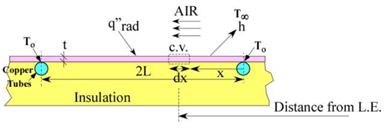4) A solar collector plate of thickness t is attached to copper tubes as shown. The collector receives combined beam and diffuse radiation at a uniform rate of . The temperature of the pipes at the point of contact to the collector maintains constant at To. The bottom surface is well insulated (i.e., no heat transfer from that side). The collector is also exposed to a moving air at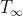with a convective heat transfer coefficient of h. Neglect any heat transfer in the direction perpendicular to the paper.

a)Write the energy balance for a typical infinitesimal control volume (c.v.) that is 1.4 meters away from the collector’s leading edge to derive a differential equation for the temperature distribution T(x) in the plate. Solve the differential equation and apply appropriate boundary conditions to determine the temperature profile. Given the following variables: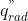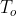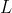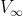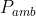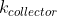Transcribed Image Text: AIR TO q"rad T. C.V. Copper Tubes 2L dx X Insulation Distance from L.E. T re T. T PA amb collector
More
Transcribed Image Text: AIR TO q"rad T. C.V. Copper Tubes 2L dx X Insulation Distance from L.E. T re T. T PA amb collector